2《数据库实战案例》-数据库sql优化总结之1-百万级数据库优化方案+案例分析(JAVA 小虚竹)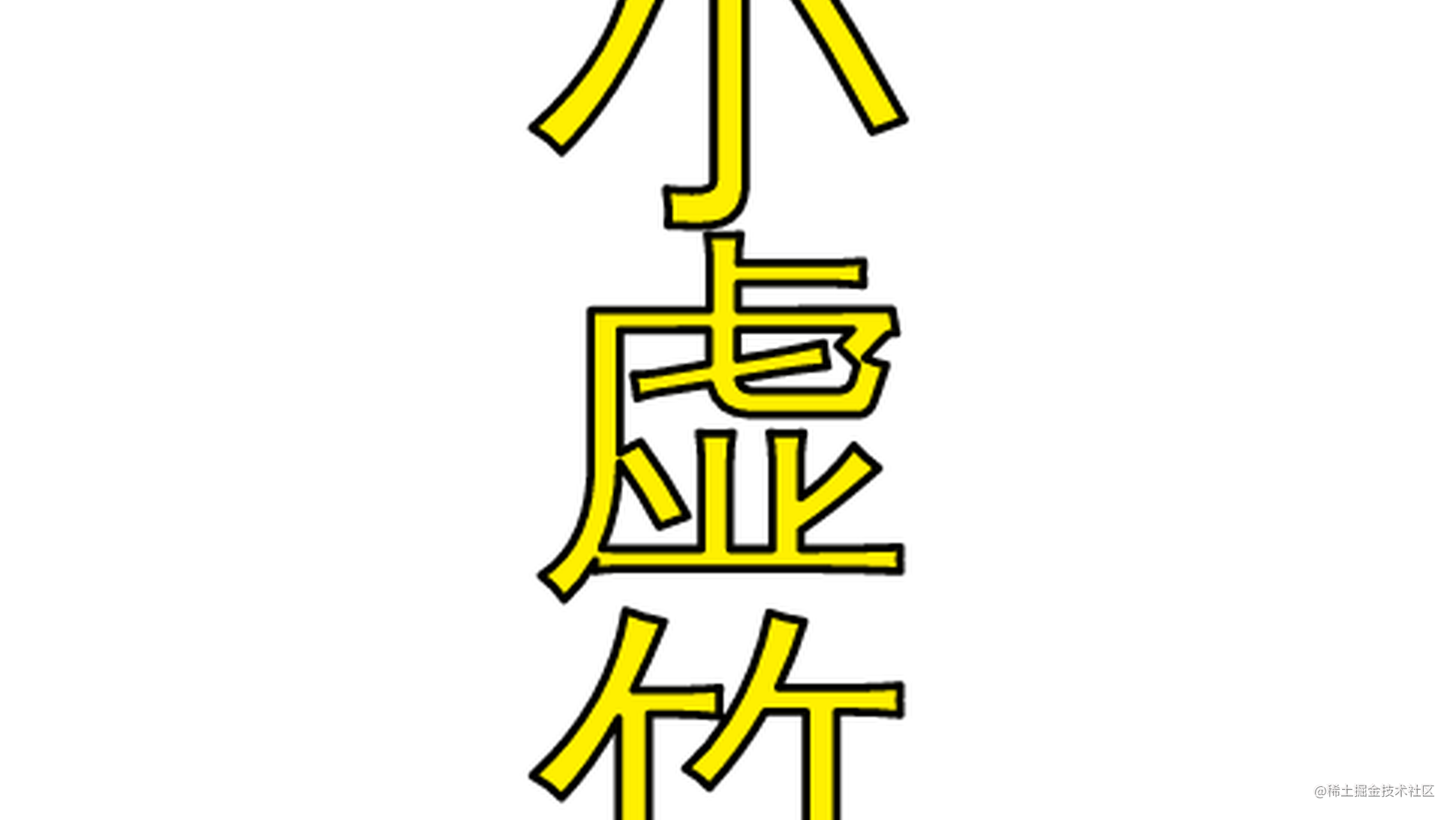❤️作者简介：大家好,我是小虚竹。Java领域优质创作者🏆，CSDN博客专家认证🏆，华为云享专家认证🏆

❤️技术活，该赏

❤️点赞 👍 收藏 ⭐再看，养成习惯

1.对查询进行优化，要尽量避免全表扫描，首先应考虑在 where 及 order by 涉及的列上建立索引。

案例分析：

SELECT ex_question_junior.QUESTION_ID
FROM ex_question_junior

执行时间：17.609s （多次执行，在17s左右徘徊）

执行时间为：11.377s（多次执行，在11s左右徘徊）

备注：我们一般在什么字段上建索引？

这是一个非常复杂的话题，需要对业务及数据充分分析后再能得出结果。主键及外键通常都要有索引，其它需要建索引的字段应满足以下条件：

a、字段出现在查询条件中，并且查询条件可以使用索引；

b、语句执行频率高，一天会有几千次以上；

c、通过字段条件可筛选的记录集很小，那数据筛选比例是多少才适合？

这个没有固定值，需要根据表数据量来评估，以下是经验公式，可用于快速评估：

小表(记录数小于10000行的表)：筛选比例<10%；

大表：(筛选返回记录数)<(表总记录数*单条记录长度)/10000/16

单条记录长度≈字段平均内容长度之和+字段数*2

以下是一些字段是否需要建B-TREE索引的经验分类：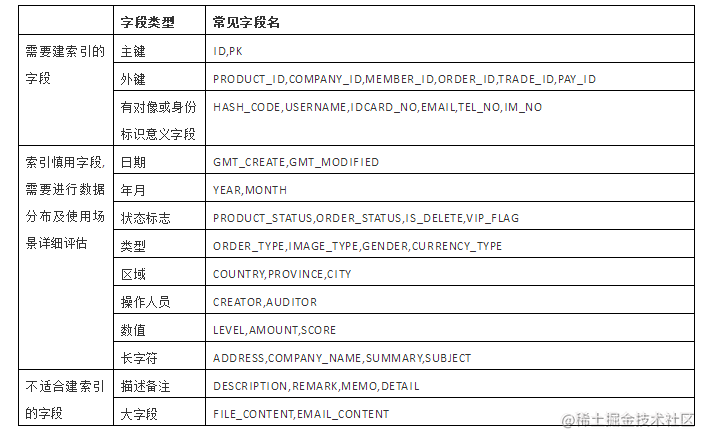2、应尽量避免在 where 子句中对字段进行 null 值判断，否则将导致引擎放弃使用索引而进行全表扫描

select id from t where num is null

select id from t where num = 0

案例分析：

SELECT ex_question_junior.QUESTION_ID
FROM ex_question_junior
WHERE IS_USE is NULL

SELECT ex_question_junior.QUESTION_ID
FROM ex_question_junior
WHERE IS_USE =0

3、应尽量避免在 where 子句中使用 != 或 <> 操作符，否则将引擎放弃使用索引而进行全表扫描。

案例分析：

EXPLAIN
SELECT ex_question_junior.QUESTION_ID
FROM ex_question_junior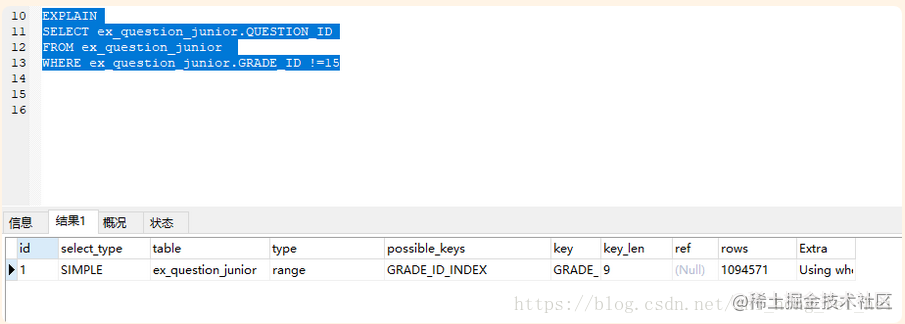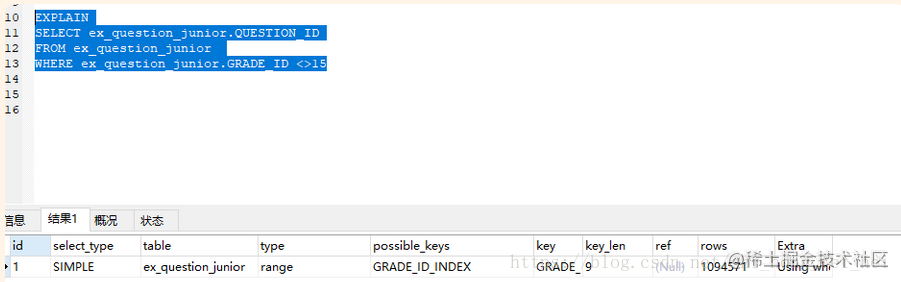4.应尽量避免在 where 子句中使用 or 来连接条件，如果一个字段有索引，一个字段没有索引，将导致引擎放弃使用索引而进行全表扫描

案例分析：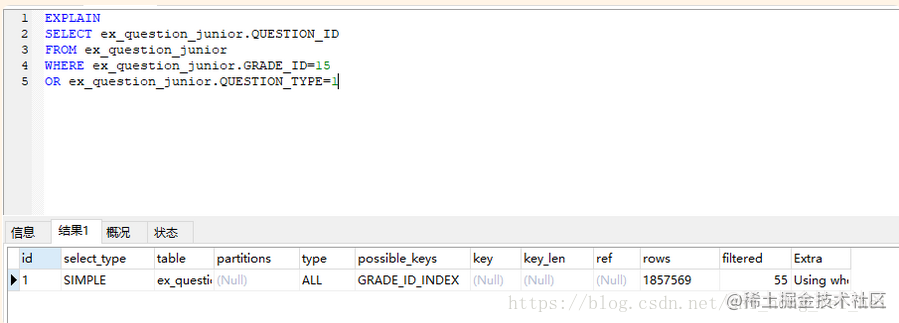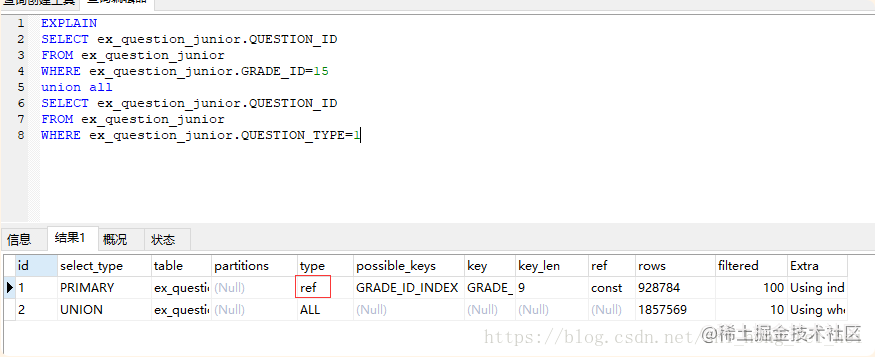5.in 和 not in 也要慎用，否则会导致全表扫描

案例分析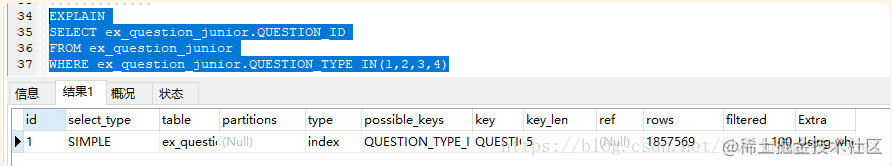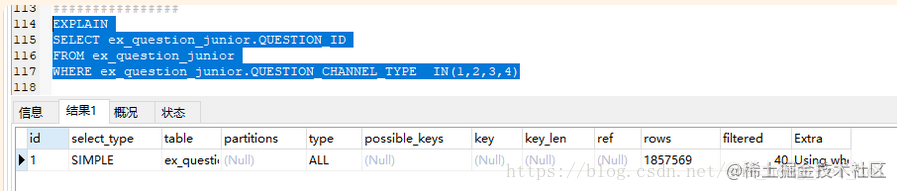案例分析2：

SELECT ex_question_junior.QUESTION_ID
FROM ex_question_junior
WHERE ex_question_junior.QUESTION_TYPE  IN(1,2,3,4)

SELECT ex_question_junior.QUESTION_ID
FROM ex_question_junior
WHERE ex_question_junior.QUESTION_TYPE between 1 and 4

案例分析3：

1. in()适合B表比A表数据大的情况（A<B）

select * from A

where id in(select id from B)

2. exists()适合B表比A表数据小的情况(A>B)

select * from A

where exists(

select 1 from B where B.id = A.id

)

3.当A表数据与B表数据一样大时,in与exists效率差不多,可任选一个使用.语法

select * from A

where id in(select id from B)

ex_question_r_knowledge表数据量大，ex_subject_point表数据量小

****************************************************************************

ex_question_r_knowledge(A)表数据量大，ex_subject_point表数据量小(B)(A>B)

SELECT *
FROM ex_question_r_knowledge
WHERE ex_question_r_knowledge.SUBJECT_POINT_ID IN
(
SELECT ex_subject_point.SUBJECT_POINT_ID
FROM ex_subject_point
WHERE ex_subject_point.SUBJECT_ID=7
)

SELECT *
FROM ex_question_r_knowledge
WHERE  exists
(
SELECT 1
FROM ex_subject_point
WHERE ex_subject_point.SUBJECT_ID=7
AND ex_subject_point.SUBJECT_POINT_ID = ex_question_r_knowledge.SUBJECT_POINT_ID
)

*************************************************************************

ex_subject_point表数据量小(A),ex_question_r_knowledge(B)表数据量大(A<B)

SELECT *
FROM ex_subject_point
WHERE
ex_subject_point.SUBJECT_POINT_ID IN( SELECT
ex_question_r_knowledge.SUBJECT_POINT_ID FROM
ex_question_r_knowledge WHERE

SELECT * FROM ex_subject_point WHERE
ex_subject_point.SUBJECT_POINT_ID IN( SELECT
ex_question_r_knowledge.SUBJECT_POINT_ID FROM
ex_question_r_knowledge WHERE

SELECT *
FROM ex_subject_point
WHERE  exists(
SELECT ex_question_r_knowledge.SUBJECT_POINT_ID
FROM ex_question_r_knowledge
AND ex_question_r_knowledge.SUBJECT_POINT_ID= ex_subject_point.SUBJECT_POINT_ID
)

6、like模糊全匹配也将导致全表扫描

案例分析

EXPLAIN
SELECT *
FROM ex_subject_point
WHERE ex_subject_point.path like "%/11/%"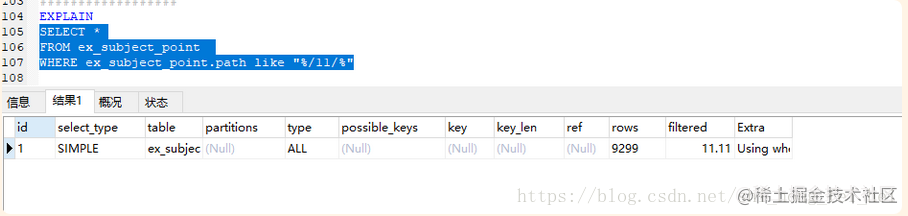like模糊后匹配，不会导致全表扫描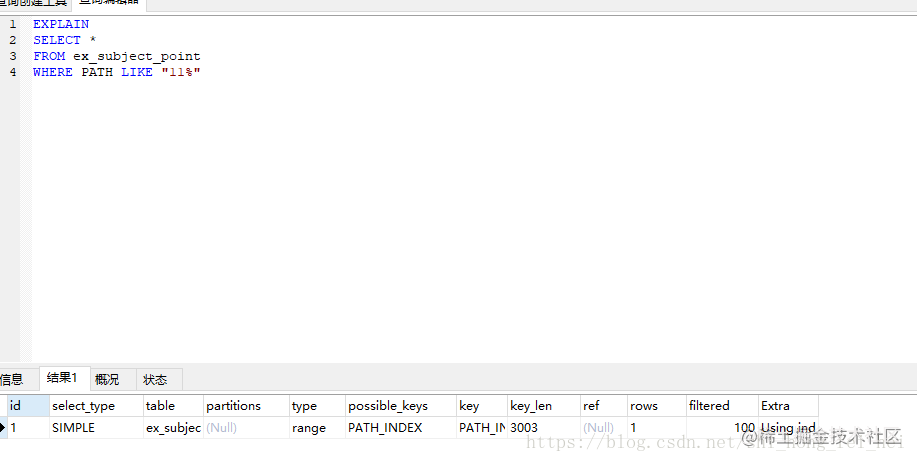like模糊前匹配，会导致全表扫描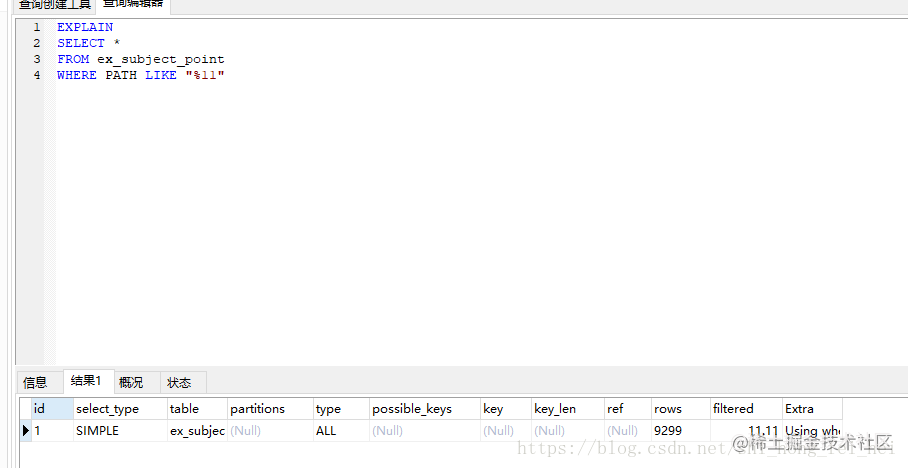MY SQL的原理就是这样的，LIKE模糊全匹配会导致索引失效，进行全表扫描；LIKE模糊前匹配也会导致索引失效，进行全表扫描；但是LIKE模糊后匹配，索引就会有效果。